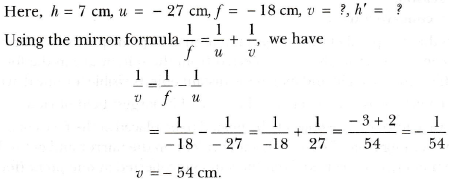3 Tutor System
Starting just at 265/hour

# An object of size 7.0 cm is placed at 27 cm in front of a concave mirror of focal length 18 cm. At what distance from the mirror should a screen be placed, so that a sharp focused image can be obtained? Find the size and the nature of the image.

Object distance, u= - 27 cm
Object height, h= 7 cm
Focal length, f = - 18 cm

According to the mirror formula,The screen should be placed at a distance of 54 cm in front of the given mirror

m = $$\frac{-54}{27}$$ = -2

Negative value indicates that image formed is real.

h' = h × m
h' = 7 × (-2) = -14 cm

The negative value of the image height indicates that the image formed is inverted.